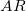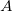# Water Application Rate

Use this form to calculate the water application rate using the flow rate onto an area. This calculator assumes perfect irrigation efficiency and uniformity. Learn more about the units used on this page.

Area:
Flow Rate onto the Area:
Water Application Rate:

## The Equation

This is the formula this calculator uses to determine the water application rate.Where:= Water application rate (in/hr)= Flow rate (gpm)= Area being irrigated (acres)

WSU Prosser - IAREC, 24106 N Bunn Rd, Prosser WA 99350-8694, 509-786-2226. Contact Us